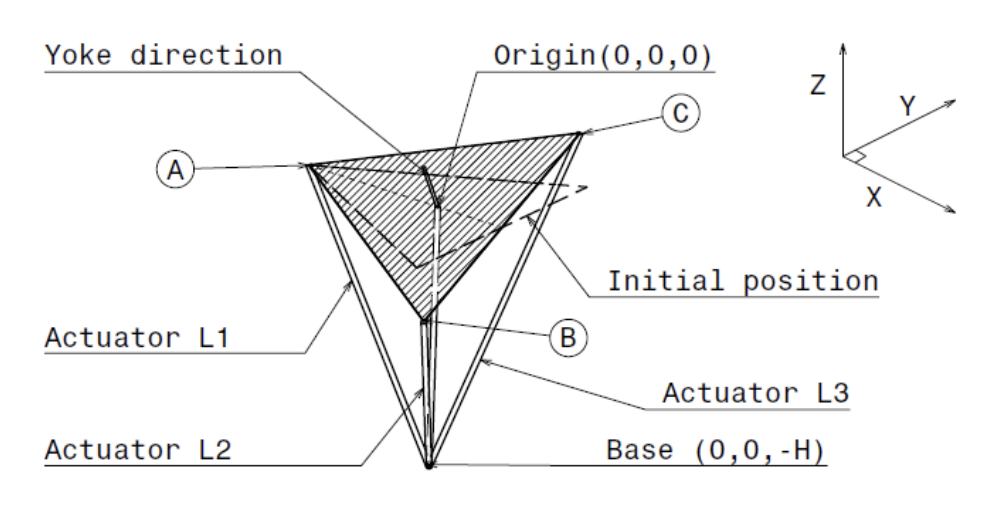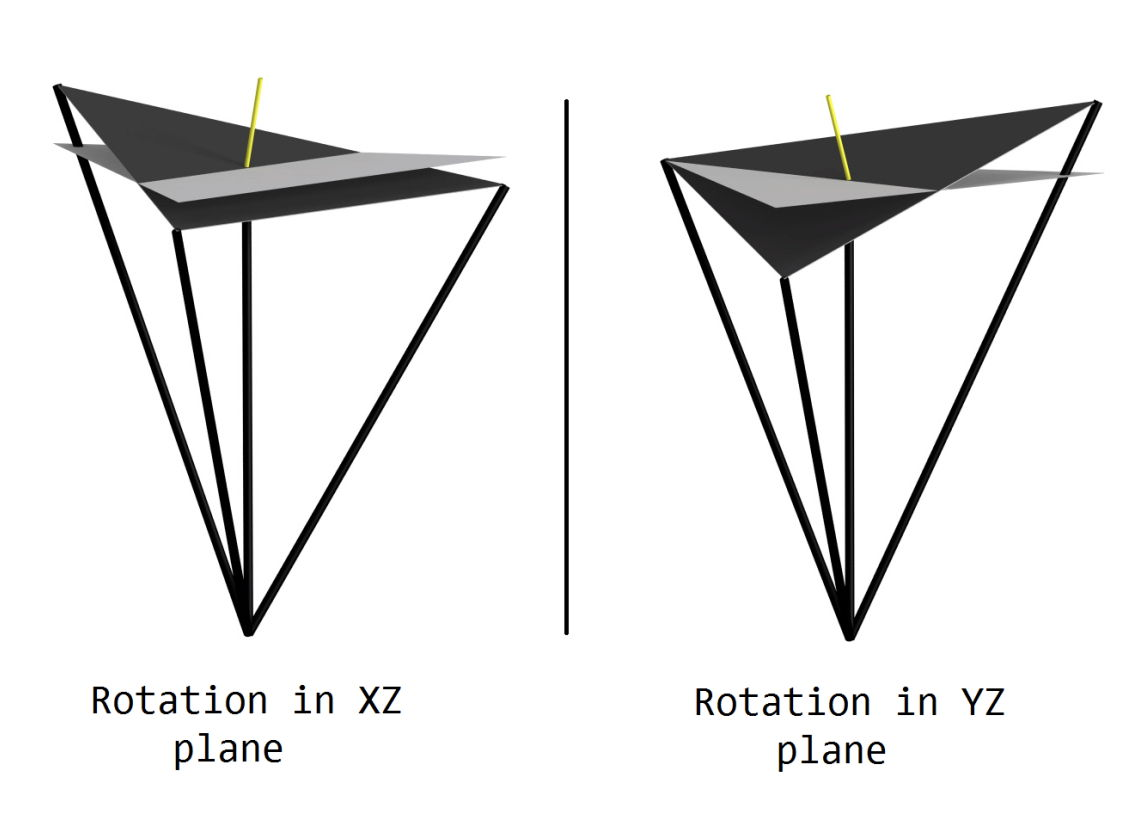시간 제한 메모리 제한 제출 정답 맞은 사람 정답 비율
6 초 512 MB 20 6 4 23.529%

## 문제

It’s the year 10, 007 AD, and all the delicious food in the Arab region has gone extinct. Luckily for you, you made once a time travel in the past and you could taste how delicious this food was. An alien rumor has come to you saying that there’s still remaining Arab food in Jupiter. Since the Alien rumors, unlike humans’, are usually true, you decided to build your own spacecraft to go to Jupiter. In order to test the control system you embedded in your spacecraft, you want to build a flight simulator, which is a device that artificially re-creates spacecraft flight and the environment in which it flies, for astronaut training and other purposes.

In this problem, a flight simulator consists of a 2-dimensional equilateral triangle of side length L, with three actuators (mechanical devices, that can be treated as line segments of modifiable lengths) attached to the vertices A, B, C of the triangle, respectively. The other ends of the actuators are all attached to a static base point located at (0, 0, −H). The midpoint of the triangle (located at (0, 0, 0)) is attached to the same base using a spherical connection, which means that the triangle can rotate in any direction but its midpoint cannot move.

The yoke (a device used to control the rotations of the triangle) has the direction vector along N = (Nx, Ny, Nz) which is always normal on the plane of the triangle. By changing the direction of the yoke, the length of each actuator changes and the triangle rotates (the yoke will remain normal on the triangle), while the midpoint remains at (0, 0, 0). Initially, all the actuators have the same length, the triangle lies horizontally in the XY plane (i.e. z = 0) and the yoke is pointing along the positive Z-axis (i.e. initially N = (0, 0, 1)). The point A is initially located on the negative X-axis (zA = yA = 0, xA < 0) and the vertices A, B, C are in an anticlockwise order relative to the yoke direction.We want you to write a program that makes the simulator rotate given a new yoke direction. Formally, given both of a yoke direction and the location of the point A after rotation, we need you to calculate the corresponding lengths of the actuators L1, L2, L3, which are attached to the vertices A, B, C respectively, so that the yoke direction is still normal on the triangle.

## 입력

The first line of the input contains a single integer T specifying the number of test cases.

Each test case consists of a single line containing eight integers Nx, Ny, Nz, Ax, Ay, Az, L, and H (−500 ≤ Ax, Ay, Az, Nx, Ny ≤ 500, 1 ≤ Nz, H, L ≤ 500), in which Nx, Ny, and Nz is the yoke direction after rotation, Ax, Ay, and Az is the position of the point A after rotation, L is the side length of the triangle, and H is the point in the base where actuators are attached

It is guaranteed that the following constraints hold for all test cases:

• Ax, Ay, Az ≠ 0
• AxNx + AyNy + AzNz = 0
• Ax2 + Ay2 + Az2 = L2/3.

## 출력

For each test case, print a single line containing three space-separated decimal numbers (rounded to exactly 6 decimal places), the lengths L1, L2, L3 of the actuators connected to the vertices A, B, C corresponding to the given query.

The given input will guarantee that there is exactly one solution.

## 예제 입력 1

2
13 -18 1 7 5 -1 15 12
-12 -17 1 7 -5 -1 15 3


## 예제 출력 1

13.964240 20.238885 7.237923
8.831761 11.473743 6.507936


## 힌트

The figures below show different positions of the flight simulator for different yoke directions :